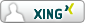Markus Wernig

UNIX/Network Security Engineer
CCSA, CCSE
CISSP

PGP key transition note
GPG Key
(in use after Aug. 9 2013)

old GPG Key
(in use up to Aug. 9 2013)

 Personal | Professional | IT related | Writings de

# How to calculate IP6.ARPA format from IPv6 addresses

## Abstract

This article shows how to check if an IPv6 address is valid with a regular expression and how to calculate the "reverse" (ie. IP6.ARPA) address query string.

This is the (Perl) regex to check if an IPv6 address is a valid interface address:

``` my \$isvalid = (((\$ip =~ s/:/:/g) <= 7) && \$ip =~ /^(::([a-f0-9]{1,4}:){0,6}|([a-f0-9]{1,4}:){7}|([a-f0-9]{1,4}:){0,7}([a-f0-9]{1,4}::){1}([a-f0-9]{1,4}:){0,7})[a-f0-9]{1,4}(%[a-z]+[0-9]+){0,1}\$/i); ```

This checks only for valid interface addresses, not network addresses in the zero-compressed form (eg. `2a00::`).

Let's have a look at the parts of the regex:

 ``` my \$isvalid = (   (     (\$ip =~ s/:/:/g) <= 7   ) &&   \$ip =~ /^   (     ::([a-f0-9]{1,4}:){0,6}   |     ([a-f0-9]{1,4}:){7}   |     ([a-f0-9]{1,4}:){0,7}     ([a-f0-9]{1,4}::){1}     ([a-f0-9]{1,4}:){0,7})   )   [a-f0-9]{1,4}   (%[a-z]+[0-9]+){0,1}\$   /i ); ``` ``` # address contains at most 7 colons # AND (ie. both conditions must match) # address starts with: # a double colon (::), followed by 0 to 6 (1-2-byte) hex# numbers, each followed by a single colon # OR address starts with: # 7 hex numbers, with trailing colon (uncompressed form) # OR address starts with: # 0 to 7 hex numbers, with trailing colon, followed by # 1 hex number, with trailing double colon, followed by # 0 to 7 hex numbers, with trailing colon # address ends with a hex number, optionally # followed by up to 1 interface identifier (for link-local) # all matches are case-insensitive ```

And here's how to calculate the "reverse" IP form from an IPv6 address (still in Perl):

``` #!/usr/bin/perl -w # small script that calculates the ip6.arpa format of an IPv6 address # public at wernig.net 2014 use strict; my (\$ip) = @ARGV or exit 1; chomp(\$ip); unless (((\$ip =~ s/:/:/g) <= 7) && \$ip =~ /^(::([a-f0-9]{1,4}:){0,6}|([a-f0-9]{1,4}:){7}|([a-f0-9]{1,4}:){0,7}([a-f0-9]{1,4}::){1}([a-f0-9]{1,4}:){0,7})[a-f0-9]{1,4}(%[a-z]+[0-9]+){0,1}\$/i) {   print "\$ip is not a valid IPv6 address\n" and exit 1; } (\$ip, undef) = split(/\%/, \$ip); # remove trailing interface identifier for link-local my @num = split(/:/, \$ip); my \$revip; my \$missing = 8 - \$#num; # how many double-octets have to be expanded? my \$exp = 0; # wether or not we have already expanded for (my \$i=\$#num; \$i>=0; \$i--) {   if(\$num[\$i] =~ /^\$/ and not \$exp) { # empty, "::"     for (my \$j=0; \$j<\$missing; \$j++) { # expand "::" with missing zeroes       \$revip .= "0.0.0.0."; # until we have right length     }     \$exp = 1; # record this, so we expand only once   }   else { # got a number string     my @lit = split(//, \$num[\$i]); # split into single chars     my \$blk ='';     for (my \$j=\$#lit; \$j>=0; \$j--) { # append single chars backwards       \$blk .= \$lit[\$j] . '.';     }     for (my \$j = 0; \$j < (3-\$#lit); \$j++) { # append "0." for every char less than 4       \$blk .= "0.";     }     \$revip .= \$blk;   } } \$revip .= "ip6.arpa"; print "Reverse IP is \$revip\n"; ```

Feel free to send any suggestions, questions and corrections to the webmaster link below (requires javascript).webmaster wernig net